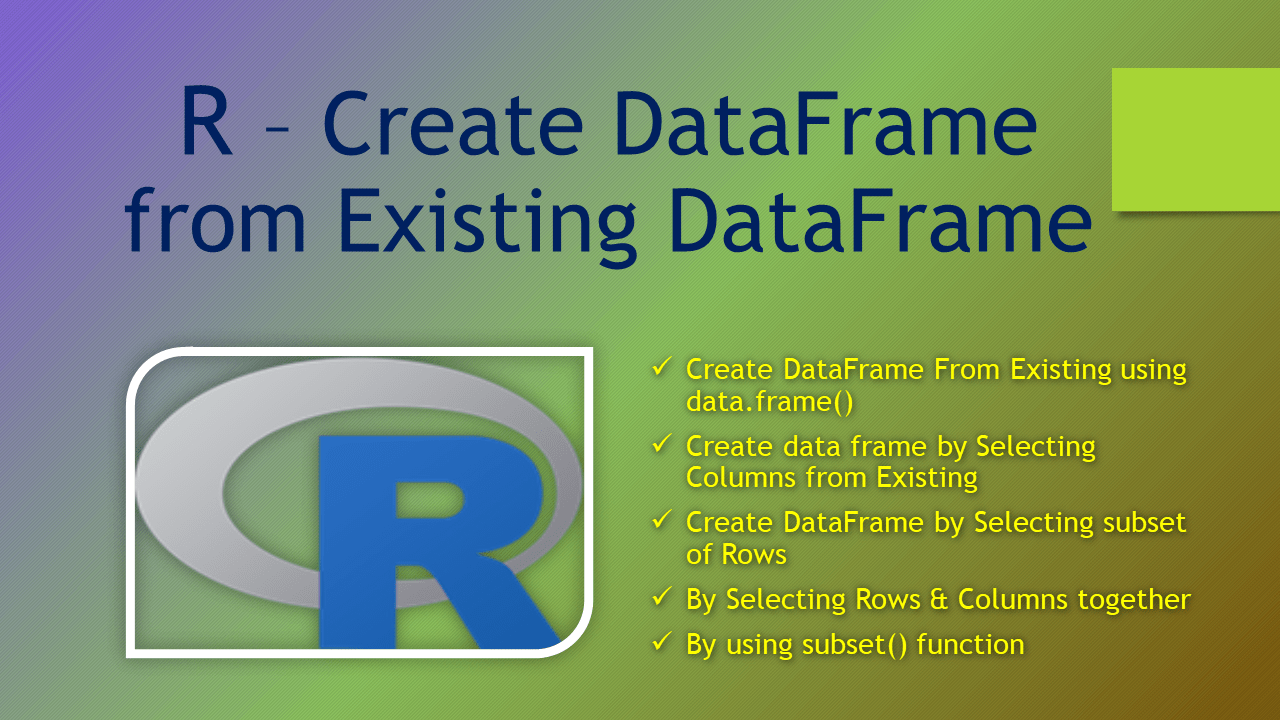# R – Create DataFrame from Existing DataFrame

You are often required to create a DataFrame from an existing DataFrame in R. When you create from an existing you may be required to select subset of columns or select only a few rows by filtering. This is one of the most use-cases when we are working with the data.

## 1. Quick Examples

The following are quick examples of how to create a DataFrame from an existing R DataFrame.

``````
# Quick examples

# Example 1 - Select columns id, gender and dob
df2 = data.frame(df\$id,df\$gender,df\$dob)

# Example 2 - Create DataFrame with 1,3 and 4 columns
df2 <- df[,c(1,3,4)]

# Example 3 - Create DataFrame with selecting range of columns
df2 <- df[,c(1:3,5)]

# Example 4 - Create DataFrame with id,gender and name columns
df2 <- df[,c('id','gender','dob')]

# Example 5 - Create DataFrame with 1,3 and 4 rows
df2 <- df[c(1,3,4),]

# Example 6 - Create DataFrame with 1,3,4 rows and columns 2 and 4
df2 <- df[c(1,3,4),c(2,4)]

# Example 7 - By using subset with column names
df2 <- subset(df, select=c("id", "gender", "dob"))

# Example 8 -  By using subset with indices
df2 <- subset(df, select=c(2:3, 5))
``````

Let’s create a DataFrame, run these examples and explore the output.

``````
# Create DataFrame
df <- data.frame(
id = c(10,11,12,13),
name = c('sai','ram','deepika','sahithi'),
gender = c('M','M','F','F'),
dob = as.Date(c('1990-10-02','1981-3-24','1987-6-14','1985-8-16')),
state = c('CA','NY','DE','FL')
)

# Print DataFrame
df

# Output
#  id    name gender        dob state
#1 10     sai      M 1990-10-02    CA
#2 11     ram      M 1981-03-24    NY
#3 12 deepika      F 1987-06-14    DE
#4 13 sahithi      F 1985-08-16    FL
``````

## 2. Create DataFrame From Existing using data.frame()

data.frame() method is used to create a DataFrame in R and also is used to create an empty DataFrame. Similarly, you can also use this to create a DataFrame by selecting subset columns and rows from an existing one.

``````
# Create DF by selecting columns id, gender and dob
df2 = data.frame(df\$id,df\$gender,df\$dob)
df2

# Output
#  df.id df.gender     df.dob
#1    10         M 1990-10-02
#2    11         M 1981-03-24
#3    12         F 1987-06-14
#4    13         F 1985-08-16
``````

Note that column names have data frame prefix if you can rename columns by using R function colnames().

## 3. Create data frame by Selecting Columns from Existing

You can also create a DataFrame by selecting columns from the existing DataFrame. While selecting the columns you can also by name, indices, and use a range of columns.

``````
# Create DataFrame with id,gender and name columns
df2 <- df[,c('id','gender','dob')]
df2

# Output
#  id gender        dob
#1 10      M 1990-10-02
#2 11      M 1981-03-24
#3 12      F 1987-06-14
#4 13      F 1985-08-16
``````

The same output can also be achieved by using indices.

``````
# Create DataFrame with 1,3 and 4 columns
df2 <- df[,c(1,3,4)]
df2
``````

Similarly, you also select columns by ranges of indices.

``````
# Create DataFrame with selecting range of columns
df2 <- df[,c(1:3,5)]
df2
``````

## 4. Create DataFrame by Selecting subset of Rows

To create a DataFrame by selecting subset of rows from the existing DataFrame use the below approach. From the following example `df[c(1,3,4),]` returns rows 1, 3, and 4.

``````
# Create DataFrame with 1,3 and 4 rows
df2 <- df[c(1,3,4),]
df2

# Output
#  id    name gender        dob state
#1 10     sai      M 1990-10-02    CA
#3 12 deepika      F 1987-06-14    DE
#4 13 sahithi      F 1985-08-16    FL
``````

## 5. By Selecting Rows & Columns together

using the same approach select the rows and columns together and initialize the DataFrame with the result.

``````
# Create DataFrame with rows 1,3 and 4 and columns 2,4
df2 <- df[c(1,3,4),c(2,4)]
df2

# Output
#     name        dob
#1     sai 1990-10-02
#3 deepika 1987-06-14
#4 sahithi 1985-08-16
``````

## 6. By using subset() function

`subset()` is a R primitive function that is used to select the columns from the DataFrame and assign this result to the variable to create df with the result.

``````
# By using subset with column names
df2 <- subset(df, select=c("id", "gender", "dob"))
df2

# Output
#  id gender        dob
#1 10      M 1990-10-02
#2 11      M 1981-03-24
#3 12      F 1987-06-14
#4 13      F 1985-08-16
``````

You can also use the `subset()` to select columns by indices.

``````
# By using subset with indices
df2 <- subset(df, select=c(2:3, 5))
df2
``````

## Conclusion

In this article, you have learned several examples of how to create a DataFrame from the existing DataFrame in R. When you create from an existing you may be required to select a subset of columns or select only a few rows by filtering. This is one of the most use-cases when we are working with the data.

## References

### Naveen (NNK)

Naveen (NNK) is a Data Engineer with 20+ years of experience in transforming data into actionable insights. Over the years, He has honed his expertise in designing, implementing, and maintaining data pipelines with frameworks like Apache Spark, PySpark, Pandas, R, Hive and Machine Learning. Naveen journey in the field of data engineering has been a continuous learning, innovation, and a strong commitment to data integrity. In this blog, he shares his experiences with the data as he come across. Follow Naveen @ @ LinkedIn